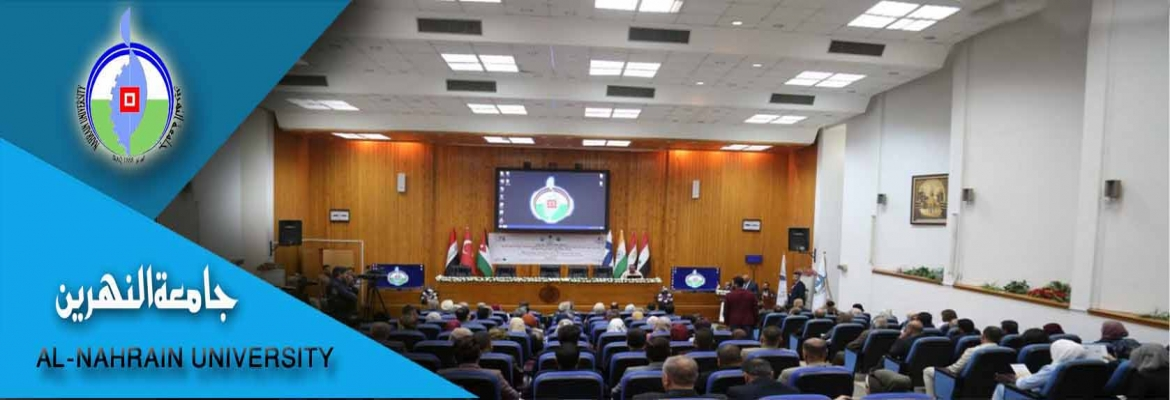# Thesis

## THE THEORETICAL INVESTIGATION OF DRAG COEFFICIENT AND SETTLING VELOCITY CORRELATIONS

The aim of this research is to find general empirical equations for predicting drag coefficient and settling velocity (for polymeric system of Car boxy Methyl cellulose CMC and Hydroxyl Ethel cellulose HEC as non-Newtonian fluids and water as Newtonian fluid), and to compare these equations with the other equations in literatures to show the accuracy of the developed equations.Empirical equation was found to relate drag coefficient C and Reynolds number Rep for a wide range of Repd from 0.001 to 10000 and for spherical and irregular shaped particles for Newtonian and non-Newtonian flu

## SIMULATION ON DYNAMIC AND CONTROL OF DISTILLATION COLUMN

The distillation column is one of the essential separation processes in modern chemical and petrochemical industries so simulation of dynamic and control of distillation column was conducted in this work. For the control system, the important controlled variables were chosen to be the distillate composition (XD), and the side stream composition (XS).

## : INFLUENCE OF SOME ADDITIVES ON PROPERTIES OF GREASES

This work aims to study the influence of some additives on the properties of soap greases, such as lithium, calcium, sodium, lithium-calcium by adding first: additives including graphite,molybdenum disulfide, carbon black, corrosion inhibiter and extreme pressure, second: base stock oils 40, 60, 150.Various additives have been added to greases to obtain the best percentages that improve the properties of grease such as load carrying, wear resistance, corrosion resistance,drop point and penetration.The result showed the best weight percentages to all types of gre

## “Experimental and kinetics of vanadium recovery from spent catalyst using caustic soda

A Theoretical  and experimental investigation was conducted to study recovery of vanadium from spent catalyst via leaching process using sodium hydroxide to investigate the effect of process variables on vanadium recovery and study kinetic of recovered  was investigated by determining the controlling step.The effect of process variables (temperature, particle size,molarity of sodium hydroxide and leaching time) on the percentages of vanadium recovery were investigated and it was found that the percentage of vanadium recovery increased with:

## STUDYING THE EFFECT OF OPERATING CONDITIONS ON WATER TREATMENT USING RO

The present study is to analyze and model concentration polarization in spiral wound brackish water membrane elements. The aim of the present work is to study the effect of different operating parameters on the reverse osmosis system which are feed flow rate, feed concentration, and feed temperature on permeate concentration and mass transfer coefficient for the brackish water system.

## Theoretical Analysis of the Optimum Bed Thickness of an Electrochemical Reactor

In this study, a theoretical analysis of optimum bed thickness for realizing a high efficiency and reaction conversion of an electrochemical reactor has been made based on flow-through porous electrode (FTPE) configuration. Another configuration of porous electrode (flow-by) has been reviewed beside the considered one. The method that has been used is to find out a mathematical model to represent the optimum bed thickness by taking a look into previous works concerned and collecting all related information, data, and models.

## Studying the Control of Absorption Column

The absorption column is one of the essential separation processes in industrial operation, so the need arises to control absorption column by process simulation and also to analyze system by method called frequency response using MATLAB8 .This work dealt with gas-liquid (air-water) absorption packed column which is analyzed by bode plot and frequency response to determine the stability of the system with or without Proportional Controller (P), Proportional Integral Controller (PI) or Proportional Integral Derivatives Controller (PID).The frequency r

## Inhibition of Galvanic Corrosion In Sodium Chloride Solutions

Because of the importance of galvanic corrosion the need arises to study the effect of corrosion inhibitors, area ratio of cathode to anode (Ac/A), on galvanic corrosion of several industrially important metals and also to follow the a behavior of corrosion rate of each member of the couple.  In the present experiments at the first stage, the corrosion potential with time was measured for four types of metals in solution of four pH values. The purpose of this stage is to find out the cathode metal from the anode.

## Investigation of some factors affecting the performance of biochemical reactor using MATLAB

In this work, it was attempted to estimate the mass a) and study the dynamic behavior of continuous stirred tank fermenter (CSTF) by means of Matlab language and benefit from commands and functions,which are built in Matlab.transfer coefficient (kL The present work developed a mathematical model for production of biomass as a single cell protein (SCP) by treating backer yeast (biomass) with glucose (substrate 1) and oxygen (substrate 2) as an example for CSTF and used in simulation n programs.

## In the theoretical part, Equilibrium (EQ) and Rate-Based or Non Equilibrium (NEQ) models were developed using MATLAB (R2009b) to solve MESHR equations, M: Material balance, E: Equilibrium relation, S: Summation equations, H: Heat balance, and R: Re

This investigation represent an experimental study which was conducted on a flow-by fixed bed electrochemical reactor in which electrolyte is perpendicular to the current flow direction.

## اخبار كلية الطب

Education - This is a contributing Drupal Theme
Design by WeebPal.